# Magnetic Inductance Flashcards

Set Details Share
created 5 years ago by ssdesa_100
2,751 views
updated 5 years ago by ssdesa_100
Page to share:
Embed this setcancel
COPY
code changes based on your size selection
Size:
X

1

The rate of change of flux has which of the following units:

B) joules
C) volts
D) m/s
E) webers

C

2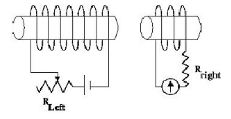For the solenoids shown in the diagram (which are assumed to be close to each other), the resistance of the left-hand circuit is slowly increased. In which direction does the ammeter needle (indicating the direction of conventional current) in the right-hand circuit deflect in response to this change?
A) The needle deflects to the left.
B) The needle deflects to the right.
C) The needle oscillates back and forth.
D) The needle rotates in counterclockwise circles.
E) The needle never moves.

A

3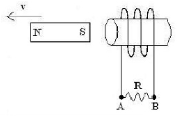A strong bar magnet is held very close to the opening of a solenoid as shown in the diagram. As the magnet is moved away from the solenoid at constant speed, what is the direction of conventional current through the resistor shown and what is the direction of the force on the magnet because of the induced current?

Current through Resistor Force on Magnet

A) From A to B To the left
B) From B to A To the left
C) From A to B To the right
D) From B to A To the right
E) No current To the right

A

4

A magnet is dropped through a vertical copper pipe slightly larger than the magnet. Relative to the speed it would fall in air, the magnet in the pipe falls.
A) more slowly because it is attracted by the innate magnetic field of the pipe
B) more slowly because the currents induced in the pipe produce an opposing magnetic field
C) at the same rate
D) more quickly because it is attracted by the innate magnetic field of the pipe
E) more quickly because the currents induced in the pipe produce an opposing magnetic field

C

5

A 0.20 m long copper rod has constant velocity 0.30 m/s traveling through a uniform magnetic field of 0.060 T.The rod, velocity, and magnetic field are all mutually perpendicular. What is the potential difference induced across the rod’s length?
A) 0.0036 V
B) 0.040 V
C) 0.090 V
D) 1.0 V
E) 25 V

B

6

When a wire moving through a magnetic field has a voltage induced between the wire’s ends, that voltage is
I. directly proportional to the strength of the magnetic field
II. directly proportional to the velocity of the wire
III. directly proportional to the diameter of the wire
A) I only B) II only C) III only D) I and II only E) II and III only

A

7

Lenz’s law concerning the direction of an induced current in a conductor by a magnetic field could be are statement of
A) Ampere’s law B) Ohm’s Law C) Tesla’s Law D) The Law of Conservation of Energy E) none of these

D

8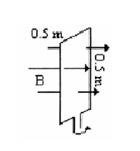A square loop is placed in a uniform magnetic field perpendicular to the plane of the loop as shown. The loop is 0.50 meters on a side and the magnetic field B has a
strength of 2 T. If the loop is rotated through an angle of 90° in 0.1 second what
would be the average induced EMF in the loop?
A) 0.025 C

B) 0.40 V

C) 5 V

D) 10 V

E) 80 V

D

9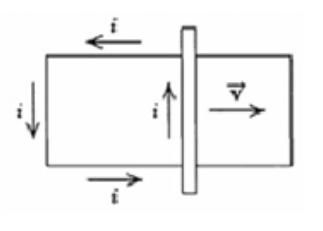The figure shows a bar moving to the right on two conducting rails. To make an induced current i in the direction indicated, in what direction would the magnetic field be in the area contained within
the conducting rails?
A) out of the page D) to the left
B) into the page E) It is impossible
C) to the right

C

10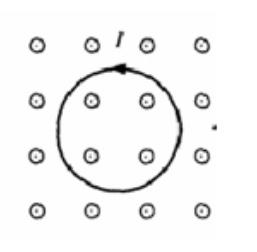There is a counterclockwise current I in a circular loop of wire situated in an external magnetic field directed out of the page as shown. The effect of the forces that act on this current is to make the loop
(A) expand in size
(B) contract in size
(C) rotate about an axis perpendicular to the page
(D) rotate about an axis in the plane of the page
(E) accelerate into the page

B

11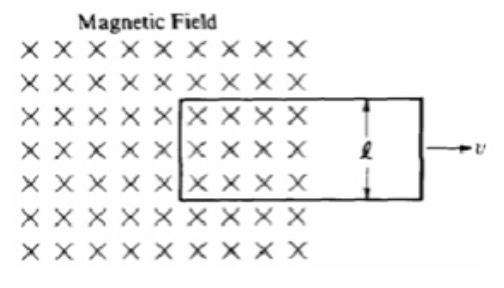The figure shows a rectangular loop of wire of width l and resistance R. One end of the loop is in a uniform magnetic field of strength B at right angles to the plane of the loop. The loop is pulled to the right at a constant speed v. What are the magnitude and direction of the induced current in the loop?
Magnitude Direction
(A) BlvR Clockwise
(B) BlvR Counterclockwise
(C) Blv/R Clockwise
(D) Blv/R Counterclockwise
(E) 0 Undefined

A

12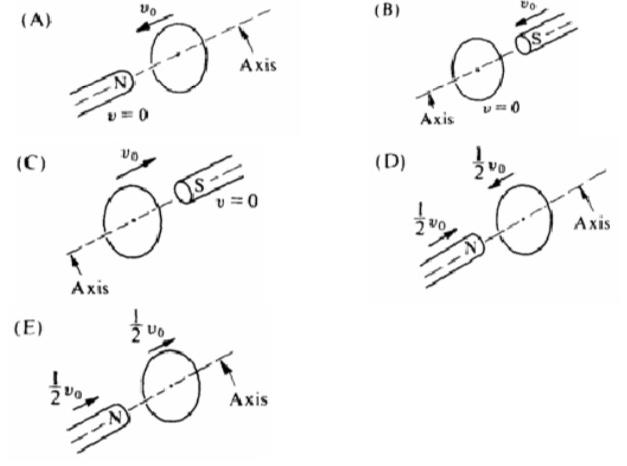In each of the following situations, a bar magnet is aligned along the axis of a conducting loop. The magnet and the loop move with the indicated velocities. In which situation will the bar magnet NOT induce a current in the conducting loop?

C

13

A square loop of copper wire is initially placed perpendicular to the lines of a constant magnetic field of 5 x 10-3 tesla. The area enclosed by the loop is 0.2 square meter. The loop is then turned through an angle of 90° so that the plane of the loop is parallel to the field lines. The turn takes 0.1 second. The average emf
induced in the loop during the turn is
(A) 1.0 x 10-4 V (B) 2.5 x 10-3 V (C) 0.01 V (D) 100 V (E) 400 V

E

14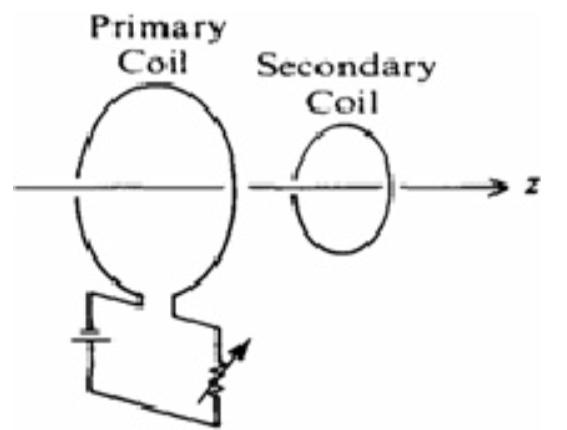Two circular coils are situated perpendicular to the z-axis as shown. There is a current in the primary coil. All of the following procedures will induce a current in the secondary coil EXCEPT
(A) rotating the secondary coil about the z-axis
(B) rotating the secondary coil about a diameter
(C) moving the secondary coil closer to the primary coil
(D) varying the current in the primary coil
(E) decreasing the cross-sectional area of the secondary coil

C

15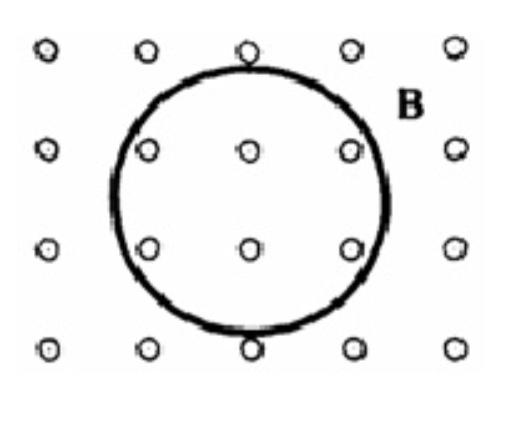A magnetic field B that is decreasing with time is directed out of the page and passes through a loop of wire in the plane of the page, as shown. Which of the following is true of the induced current in the wire loop?
(A) It is counterclockwise in direction.
(B) It is clockwise in direction.
(C) It is directed into the page.
(D) It is directed out of the page.
(E) It is zero in magnitude.

A

16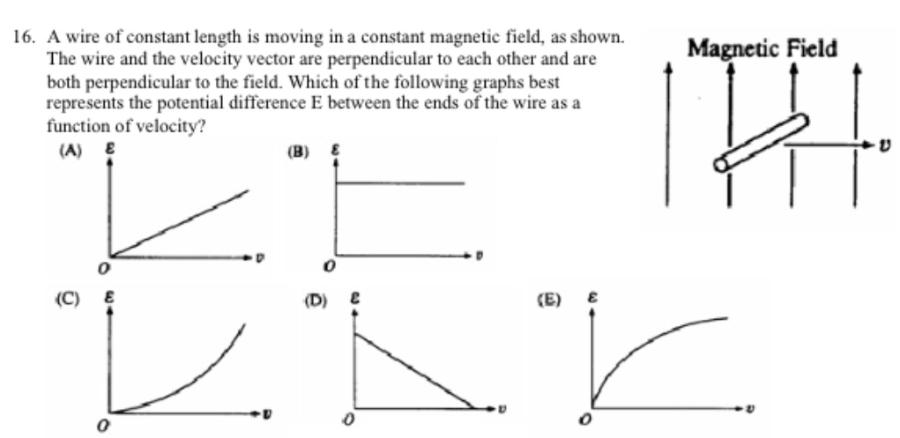A wire of constant length is moving in a constant magnetic field, as shown. The wire and the velocity vector are perpendicular to each other and are both perpendicular to the field. Which of the following graphs best represents the potential difference E between the ends of the wire as a function of velocity?

A

17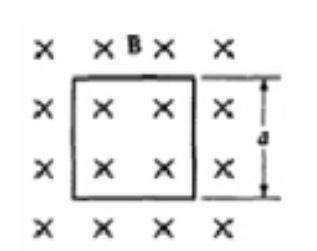A square loop of wire of resistance R and side a is oriented with its plane perpendicular to a magnetic field B, as shown. What must be the rate of change of the magnetic field in order to produce a current I in the loop?(A) IR/a2 (B) Ia2 /R (C) Ia/R (D) Ra/I (E) IRa

A

18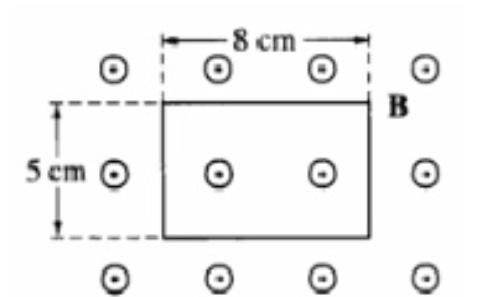A rectangular wire loop is at rest in a uniform magnetic field B of magnitude 2 T that is directed out of the page. The loop measures 5 cm by 8 cm, and the plane of the loop is perpendicular to the field, as shown. The total magnetic flux through the loop is
(A) zero
(B) 2 x 10-3 T-m2
(C) 8 x 10-3 T-m2
(D) 2 x 10-1 T-m2
(E) 8 x 10-1 T-m

A

19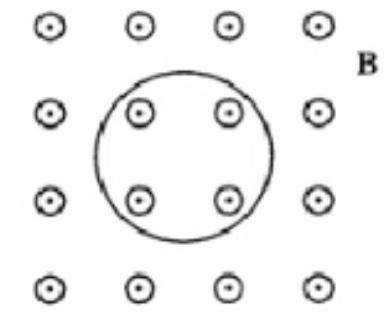A single circular loop of wire in the plane of the page is perpendicular to a uniform magnetic field B directed out of the page, as shown. If the magnitude of the magnetic field is decreasing, then the induced current in the wire is
(A) directed out of the paper
(B) directed into the paper
(C) clockwise around the loop
(D) counterclockwise around the loop
(E) zero (no current is induced)

C

20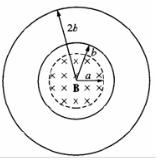A uniform magnetic field B that is perpendicular to the plane of the page now passes through the loops, as shown. The field is confined to a region of radius a, where a < b, and is changing at a constant rate. The induced emf in the wire loop of radius b is ε. What is the induced emf in the wire loop of radius 2b ?(A) Zero (B)ε/2 (C)ε (D) 2ε (E) 4ε

D

21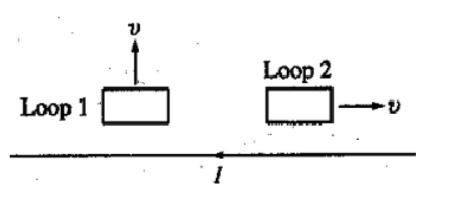Two conducting wire loops move near a very long, straight conducting wire that carries a current I. When the loops are in the positions shown, they are moving in the direction shown with the same constant speed v. Assume that the loops are far enough apart that they do not affect each other. Which of the following is true about the induced electric currents, if any, in the loops?
Loop 1 | Loop 2
(A) No Current | No Current
(B) No Current | Counterclockwise Direction
(C) Clockwise Direction | No Current
(D) Clockwise Direction | Clockwise Direction
(E) Counterclockwise Direction | Clockwise Direction

C

22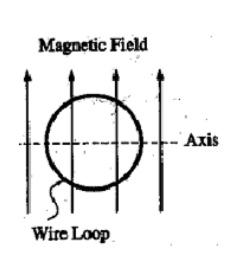A wire loop is rotated in a uniform magnetic field about an axis perpendicular to the field, as shown. How many times is the induced current in the loop reversed if the loop makes 3 complete revolutions from the position shown?(A) One (B) Two C) Three (D) Six (E) Twelve

C

23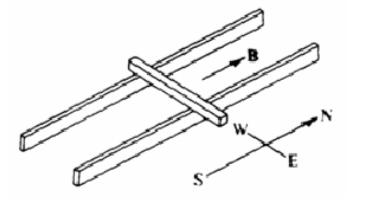The ends of a metal bar rest on two horizontal north-south rails as shown. The bar may slide without friction freely with its length horizontal and lying east and west as shown. There is a magnetic field parallel to the rails and directed north.

If the bar is pushed northward on the rails, the electromotive force induced in the bar as a result of the magnetic field will
(A) be directed upward
(B) be zero
(C) produce a westward current
(D) produce an eastward current
(E) stop the motion of the bar

D

24The ends of a metal bar rest on two horizontal north-south rails as shown. The bar may slide without friction freely with its length horizontal and lying east and west as shown. There is a magnetic field parallel to the rails and directed north.

A battery is connected between the rails and causes the electrons in the bar to drift to the east. The resulting magnetic force on the bar is directed (A) north (B) south (C) east (D) west (E) vertically

B

25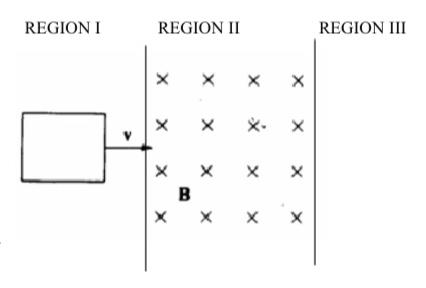A loop of wire is pulled with constant velocity v to the right through a region of space where there is a uniform magnetic field B directed into the page, as shown. The induced current is as follows
A) Directed CW both entering and leaving REGION II.
B) Directed CCW both entering and leaving REGION II.
C) Directed CW entering REGION II and CCW leaving REGION II.
D) Directed CCW entering REGION II and CW leaving REGION II.
E) zero at all times

D

26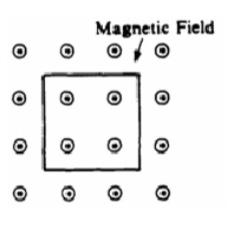A square loop of wire of side 0.5 meter and resistance 10-2 ohm is located in a uniform magnetic field of intensity 0.4 tesla directed out of the page as shown. The magnitude of the field is decreased to zero at a constant rate in 2 seconds. As the field is decreased, what are the magnitude and direction of the current in the loop?
(A) Zero
(B) 5 A, counterclockwise
(C) 5 A, clockwise
(D) 20 A, counterclockwise
(E) 20 A, clockwise

B

27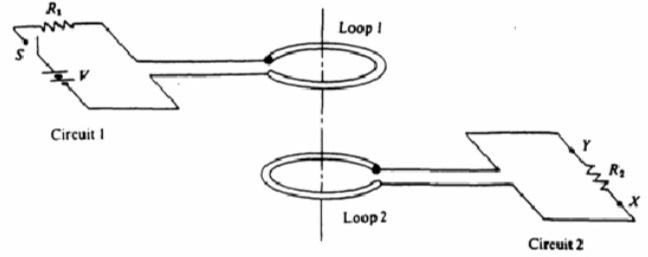After the switch S is closed, the initial current through resistor R2 is:
(A) from point X to point Y
(B) from point Y to point X
(C) zero at all times (D) oscillating between X and Y
(E) impossible to determine its direction

A

28After the switch S has been closed for a very long time, the currents in the two circuits are
(A) zero in both circuits
(B) zero in circuit 1 and V/R2 in circuit 2
(C) V/R in circuit 1 and zero in circuit 2
(D) V/R1 in circuit 1 and V/R2 in circuit 2
(E) oscillating with constant amplitude in both circuits

C

29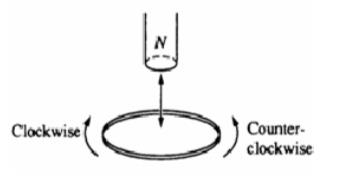In the figure, the north pole of the magnet is first moved down toward the loop of wire, then withdrawn upward. As viewed from above, the induced current in the loop is
A) always clockwise with increasing magnitude
B) always clockwise with decreasing magnitude
C) always counterclockwise with increasing magnitude
D) always counterclockwise with decreasing magnitude
E) first counterclockwise, then clockwise

E

30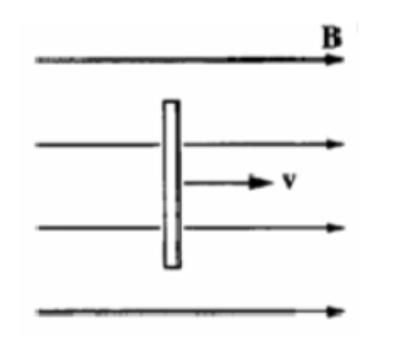A vertical length of copper wire moves to the right with a steady velocity v in the direction of a constant horizontal magnetic field B as shown. Which of the following describes the induced charges on the ends of the wire?
Top End | Bottom End
(A) Positive | Negative
(B) Negative | Positive
(C) Negative | Zero
(D) Zero | Negative
(E) Zero | Zero

E

31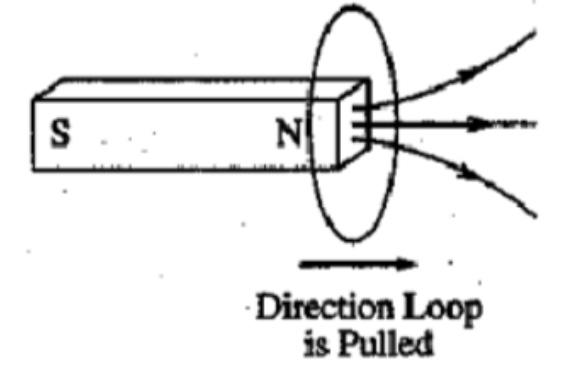A conducting loop of wire that is initially around a magnet is pulled away from the magnet to the right, as indicated in the figure, inducing a current in the loop. What is the direction of the force on the magnet and the direction of the magnetic field at the center of the loop due to the induced current?
Direction of Force on Magnet | Direction of Magnetic Field at Center of Loop due to Induced Current
(A) To the right | To the right
(B) To the right | To the left
(C) To the left | To the right
(D) To the left | To the left
(E) No direction; force is zero | To the left

A

32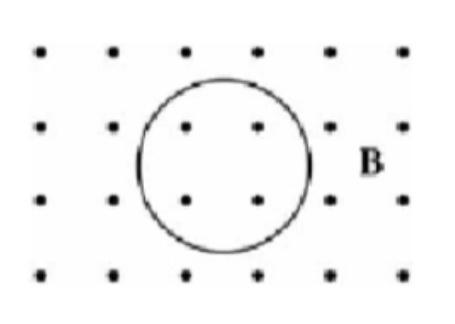A uniform magnetic field B is directed out of the page, as shown above.A loop of wire of area 0.40 m2 is in the plane of the page. At a certain instant the field has a magnitude of 3.0 T and is decreasing at the rate of 0.50 T/s. The magnitude of the induced emf in the wire loop at this instant is most nearly:
(A) 0.20 V (B) 0.60 V (C) 1.2 V (D) 1.5 V (E) 2.8 V

A

33

A large parallel-plate capacitor is being charged and the magnitude of the electric field between the plates of the capacitor is increasing at the rate dE/dt. Which of the following statements is correct about the magnetic field in the region between the plates of the charging capacitor
A) It is parallel to the electric field.
B) Its magnitude is directly proportional to dE/dt.
C) Its magnitude is inversely proportional to dE/dt.
D) Nothing about the field can be determined unless the charging current is known.
E) Nothing about the field can be determined unless the instantaneous electric field is known.

B

34What is the instantaneous current at point X immediately after the switch is closed?
A) 0 B) ℰ/R C)ℰ/2R D)ℰ/RL E)ℰL/2R

A

35When the switch has been closed for a long time what is the energy stored in the inductor?
A) Lℰ/2R
B) Lℰ2/2R2
C) Lℰ2/4R2
D) LR2/2ℰ2
E) ℰ2R2/4L

B

36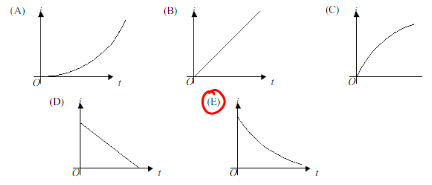After the switch has been closed for a long time, it is opened at time t=0. Which of the following graphs best represents the subsequent current i at point X as a function of time t?

E

37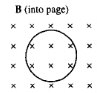A wire loop of area A is placed in a time-varying but spatially uniform magnetic field that is perpendicular to the plane of the loop, as shown above. The induced emf in the loop is given by E = b At1/2 , where b is a constant. The time varying magnetic field could be given by:
(A) 1/2bAt- 1/2
(B) 1/2bt- 1/2
(C) 1/2bt1/2
(D) 2/3bAt3 /2
(E) 2/3bt3 /2

E

38

A circular current-carrying loop lies so that the plane of the loop is perpendicular to a constant magnetic field of strength B. Suppose that the radius R of the loop could be made to increase with time t so that R = at, where a is a constant. What is the magnitude of the emf that would be generated around the loop as a function of t ?(A) 2πBa2t
(B) 2πBat
(C) 2πBt
(D) πBa2t
(E) (π/3)Ba2t3

A

39

One of Maxwell's equations can be written as ∮ E • ds = −dφ/dt. This equation expresses the fact that:
(A) a changing magnetic field produces an electric field
(B) a changing electric field produces a magnetic field
(C) the net magnetic flux through a closed surface depends on the current inside
(D) the net electric flux through a closed surface depends on the charge inside
(E) electric charge is conserved

A

40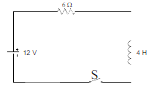What is the current in the circuit after the switch has been closed a long time?(A) 0 A (B) 1.2 A (C) 2 A (D) 3 A (E) 12 A

C

41What is the potential difference across the resistor immediately after the switch is closed?(A) 0 V (B) 2 V (C) 7.2 V (D) 8 V (E) 12 V

A

42

Two long, parallel wires, fixed in space, carry currents I1 and I2. The force of attraction has magnitude F. What currents will give an attractive force of magnitude 4F?

(A) 2I1 and 1/2I2

(B) I1 and 1/4I2

(C) 1/2I1 and 1/2I2

(D) 2I1 and 2I2

(E) 4I1 and 4I2

(D)

43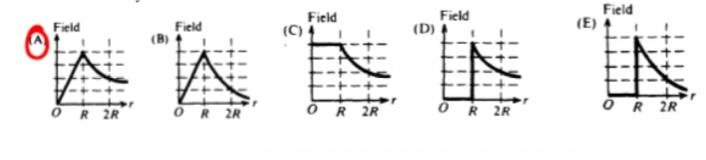A solid cylindrical conductor of radius R carries a current I uniformly distributed throughout its interior. Which of the following graphs best represents the magnetic field intensity as a function of r, the radial distance from the axis of the cylinder

(A)

44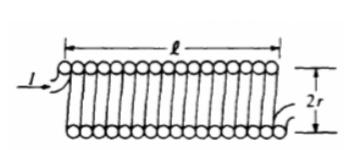The cross section above shows a long solenoid of length l and radius r consisting of N closely wound turns of wire. When the current in the wire is I, the magnetic field within this solenoid has magnitude Bo (A) B. A solenoid with the same number of turns N, length l, current I, but with radius 2r, would have a magnetic field with magnitude most nearly

(A) B0/4

(B) B0/2

(C) B0

(D) 2B0

(E) 4B0

(C)

45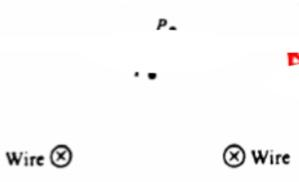Two very long parallel wires carry equal currents in the same direction into the page, as shown above. At point P, which is 10 centimeters from each wire, the magnetic field is

(A) zero

(B) directed into the page

(C) directed out of the page

(D) directed to the left

(E) directed to the right

(E)

46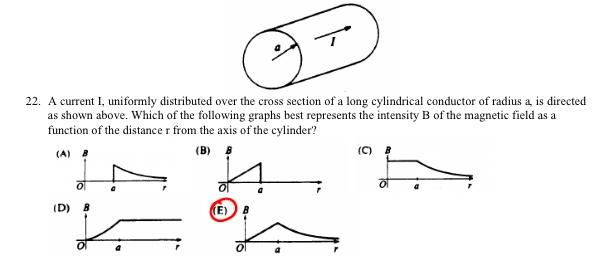A current I, uniformly distributed over the cross section of a long cylindrical conductor of radius a, is directed as shown above. Which of the following graphs best represents the intensity B of the magnetic field as a function of the distance r from the axis of the cylinder?

(E)

47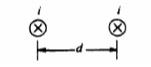Relate to the two long parallel wires shown below. Initially the wires arc a distance d apart and each has a current i directed into the page. The force per unit length on each wire has magnitude F0.

The direction of the force on the right-hand wire due to the current in the left-hand wire is

(A) to the right

(B) to the left

(C) upward in the plane of the page

(D) downward in the plane of the page

(E) into the page

(E)

48Relate to the two long parallel wires shown below. Initially the wires arc a distance d apart and each has a current i directed into the page. The force per unit length on each wire has magnitude F0.

The wires are moved apart to a separation 2d and the current in each wire is increased to 2i. The new force per unit length on each wire is:

(A) F0/4

(B) F0/2

(C) F0

(D) 2F0

(E) 4F0

(D)

49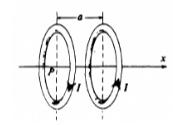The two rings each carry a current I, but in opposite directions. At point P, the center of the ring on the left the magnetic field due to these currents is

(A) zero

(B) in the plane perpendicular to the x-axis

(C) directed in the positive x-direction

(D) directed in the negative x-direction

(E) none of the above

(C)

50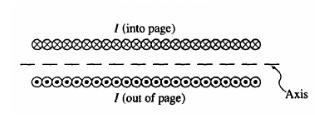A cross section of a long solenoid that carries current I is shown above. All of the following statements about the magnetic field B inside the solenoid are correct EXCEPT:

A) B is directed to the left.

B) An approximate value for the magnitude of B may be determined by using Ampere's law.

C) The magnitude of B is proportional to the current I.

D) The magnitude of B is proportional to the number of turns of wire per unit length.

E) The magnitude of B is proportional to the distance from the axis of the solenoid

(E)

51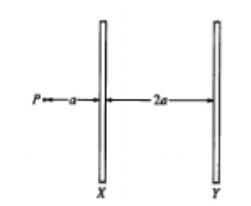Two long parallel wires are a distance 2a apart, as shown above. Point P is in the plane of the wires and a distance a from wire X. When there is a current I in wire X and no current in wire Y, the magnitude of the magnetic field at P is Bo. When there are equal currents I in the same direction in both wires, the magnitude of the magnetic field at P is

A) 2Bo/3

B) Bo

C) 10Bo/9

D) 4Bo/3

E) 2Bo

(D)

52

A narrow beam of protons produces a current of 1.6 x 10-3 A. There are 10^9 protons in each meter along the beam.

Of the following, which is the best estimate of the average speed of the protons in the beam?

(A) 10-15 m/s

(B) 10-12 m/s

(C) 10-7 m/s

(D) 10^7 m/s

(E) 10^12 m/s

(D)

53

A narrow beam of protons produces a current of 1.6 x 10-3 A. There are 10^9 protons in each meter along the beam.

Which of the following describes the lines of magnetic field in the vicinity of the beam due to the beam's current?

(A) Concentric circles around the beam

(B) Parallel to the beam

(C) Radial and toward the beam

(D) Radial and away from the beam

(E) There is no magnetic field

(A)

54A rigid, rectangular wire loop ABCD carrying current I1 lies in the plane of the page above a very long wire carrying current I2:

(A) toward the wire

(B) away from the wire

(C) toward the left

(D) toward the right

(E) zero

(A)

55

In which of the following cases does there exist a nonzero magnetic field that can be conveniently determined by using Ampere's law?

(A) Outside a point charge that is at rest

(B) Inside a stationary cylinder carrying a uniformly distributed charge

(C) Inside a very long current-carrying solenoid

(D) At the center of a current-carrying loop of wire

(E) Outside a square current-carrying loop of wire

(C)

56

A wire of radius R has a current I uniformly distributed across its cross-sectional area. Ampere's law is used with a concentric circular path of radius r, with r < R, to calculate the magnitude of the magnetic field B at a distance r from the center of the wire. Which of the following equations results from a correct application of Ampere's law to this situation?

(A) B(2πr ) = μ0 I

(B) B(2πr ) = μ0 I(r 2 /R2 )

(C) B( 2πr) = 0

(D) B( 2π R) = μ0 I

(E) B( 2π R) = μ0 I(r 2 /R 2)

(B)

57

Two parallel wires, each carrying a current I, repel each other with a force F.

If both currents are doubled, the force of repulsion is

A) 2F

(B) 2√2 F

(C) 4F

(D) 4√2 F

(E) 8F

(C)

58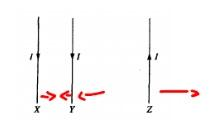The currents in three parallel wires, X, Y, and Z, each have magnitude l and are in the directions shown above. Wire Y is closer to wire X than to wire Z. The magnetic force on wire Y is

(A) zero

(B) into the page

(C) out of the page

(D) toward the bottom of the page

(E) toward the left

(E)